# IBPS Clerk Mains 2017 – Quantitative Aptitude DAY 7

Dear Banking Aspirants,

IBPS CLERK Mains 2017, the last exam of the year 2017 is going to held in 21.01.2018.There is only few days in our hands for preparation and you have to work hard to achieve your goal, because the competition is very high as per the vacancy is concerned.

So start your preparation now with this study Planner for IBPS Clerk Mains. It’s a 20 days complete planner with various Aptitude, Reasoning, English, Current affairs & Static Gk topics Included. There are 4 Mock tests available in this planner.

We have planned this Planner in Such a way that this will cover the entire current affairs quiz from the month of October to January (1st week). Because Banking Awareness, Current Affairs, Static Gk & Computer Awareness will play a vital role in cracking the IBPS Clerk Mains exam. Though the exam is getting tougher and tougher for each and every Year, Only consistently hard working candidates will crack the exam. So be a continuous hard worker and grab the opportunity to achieve your success.

Make hay while the Sunshine!!!!!!!!!!!

So as per the planner here is content related to Quantitative Aptitude .

IBPS CLERK Mains 2017 -Quantitative Aptitude DAY 7

Quantitative Aptitude

Time: 20 Minutes

D.1-5) In each of these questions, two equations (I) and (II) are given. You have to solve both the equations and give the answer.

a) x > y

b) x < y

c) x ≥ y

d) x ≤ y

e) x = y or relation cannot be established between ‘x’ and ‘y’.

Q.1) I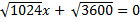II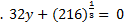Q.2)

I.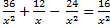II.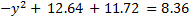Q.3)

I.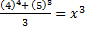II.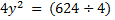Q.4)

I.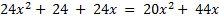II.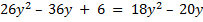Q.5)

I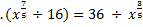IID.6-10) In each of these questions, two equations (I) and (II) are given. You have to solve both the equations and give answer.

Q.6) I.6x^2-7x-33=0

II.2y^2+11y+15=0

a) x > y

b) x < y

c) x ≥ y

d) x ≤ y

e) x = y or relation cannot be established between ‘x’ and ‘y’.

Q.7) I.x^2+15x-450=0

II.y^2-26y+168=0

a) x > y

b) x < y

c) x ≥ y

d) x ≤ y

e) x = y or relation cannot be established between ‘x’ and ‘y’.

Q.8) I.x^2-36x+299=0

II.y^2-34y+264=0

a) x > y

b) x < y

c) x ≥ y

d) x ≤ y

e) x = y or relation cannot be established between ‘x’ and ‘y’.

Q.9) I.x^2=484

y=√484

a) x > y

b) x < y

c) x ≥ y

d) x ≤ y

e) x = y or relation cannot be established between ‘x’ and ‘y’.

Q.10) I.x^2+21x+68=0

II.y^2+38y+360=0

a) x > y

b) x < y

c) x ≥ y

d) x ≤ y

e) x = y or relation cannot be established between ‘x’ and ‘y’.

Q.11)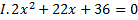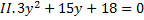a)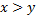b)c)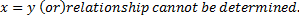d)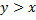e)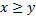Q.12)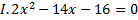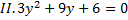a)b)c)d)e)Q.13)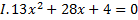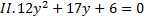a)b)c)d)e)Q.14)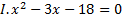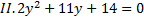a)b)c)d)e)Q.15)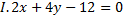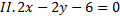a)b)c)d)e)D 16-20) Solve the equations and give the relationship between x and y.

a)b)c)d)e) x=y or Relationship can’t be determined

Q.16)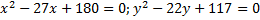Q.17)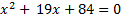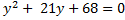Q.18)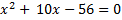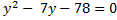Q.19)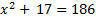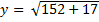Q.20)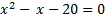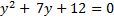Q.1) b

From I:

√1024 x+ √3600=0

=>32x+60=0

=>x=-60/32=-15/8

From II:

32y+(216)^(1/3)= 0

=>32y=-6

=>y=-6/32

From I and II, x<y

Q.2) e

36/x^2 +12/x-  24/x^2 =16/x^2

=>36/x^2 +12x/x^2 -24/x^2 -16/x^2 =0

=>(36-40)/x^2 +12x/x^2 =0

=>-4/x^2 =-12x/x^2

=>12x=4=>x=1/3

From II:

〖-y〗^2+ 12.64 +11.72 =8.36

y^2=16=>y=±4

From I and II, x and y relationship cannot be determined

Q.3) e

From I:

((4)^4+ (5)^3)/3=x^3

(256+125)/3=x^3

=>381/3=x^3=>x^3=127=>x≈5

From II:

4y^2  = (624÷ 4)

4y^2=156=>y^2=39=>y≈±6

From I and II, x and y relationship cannot be established

Q.4) a

From I:

24x^2+ 24 + 24x = 20x^2+ 44x

4x^2-20x+24=0

=>x^2-5x+6=0

x=2,3

From II:

26y^2  – 36y + 6 = 18y^2  – 20y

8y^2-16y+6=0

=>4y^2-8y+3=0

y=2/4,6/4=>1/2,3/2=>0.5,1.5

From I and II, x>y

Q.5) d

From I:

〖(x〗^(7/5)  ÷16)=36÷ x^(3/5)

=>x^(7/5)/16=36/x^(3/5) =>x^(10/5)=16×36=>x^2=576=>x=±24

From II:

y^(1/4)  ×y^(1/4)  ×7=168 ÷ y^(1/2)

=>y^(1/2)×y^(1/2)=168/7=>y=24

From I and II, x≤y

Q.6) a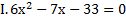-198

-18/6      11/6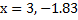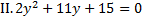30

6/2        5/2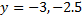x>y

Q.7) e

x^2+15x-450=0

-450

30         -15

x=-30, 15

y^2-26y+168=0

168

-14       -12

y=14, 12

Relationship cannot be determined

Q.8) e

X^2-36x+299=0

299

-13       -23

x= 13, 23

y^2-34y+264=0

264

-12         -22

y=12, 22

Relationship cannot be determined.

Q.9) d

x^2=484

x=+(or)- 22

y= Square root of 484

y=22

x ≤ y

Q.10) a

x^2+21x+68=0

68

17         4

x=-17,-4

y^2+38y+360=0

360

18          20

y=-18, -20

x>y

Q.11) c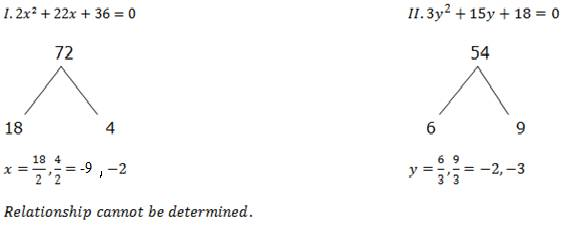Q.12) e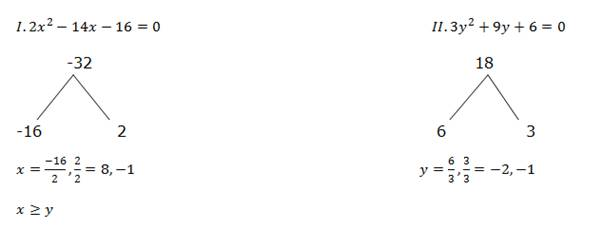Q.13) e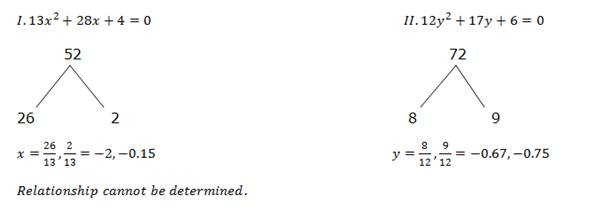Q.14) e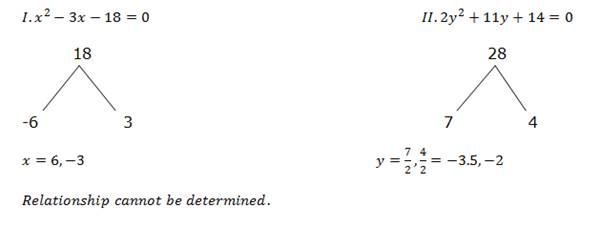Q.15) a

2x+4y-12=0——–1

2x-2y-6=0———2

Solve the above equation and we get X and Y value.

x=4, Y=1

Q.16)e

x^2-27x+180=0

x^2-12x-15x+180=0

(x-12)(x-15)

y^2-22y+117=0

(y-13)(y-9)

(y-13)(y-9)

y=13,9

Relationship can’t be determined.

Q.17)e

x^2+19x+84=(x+12)(x+7)

x=-12,-17

y^2+21y+68=(y+17)(y+4)

y=-17,-4

Relationship can’t be determined

Q.18)e

x^2+10x-56=(x+14)(x-4)

x=-14,4

y^2-7y-78=(y-13)(y+6)

y=13,-6

Relationship can’t be determined

Q.19)d

x^2+17=186

x^2=169

x=+(or)- 13

y=square root of (152+17)

y=square root of (169)

y=13

x ≤ y

Q.20)e

X^2-x-20=(x-5)(x+4)

x=5,-4

y^2+7y+12=(y+3)(y+4)

y=-3,-4

Relationship can’t be determined

You can join in our Telegram Channel the invite given below and you can share this with your friends and other aspirants who are preparing for their exams like you.

Caution: You should have Telegram app installed in your mobile or laptop to join the group. If you don’t have telegram app in your mobile. First install the app and click the link given below….